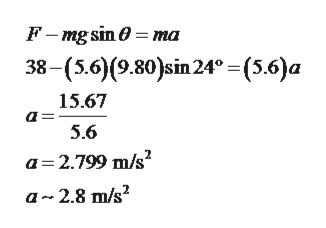# A block of mass m = 5.6 kg is pulled up a θ = 24° incline as in the figure below with a force of magnitude F = 38 N.(a) Find the acceleration of the block if the incline is frictionless. (Give the magnitude of the acceleration.)(b) Find the acceleration of the block if the coefficient of kinetic friction between the block and incline is 0.11. (Give the magnitude of the acceleration.)

Question
35 views

A block of mass m = 5.6 kg is pulled up a θ = 24° incline as in the figure below with a force of magnitude F = 38 N.

(a) Find the acceleration of the block if the incline is frictionless. (Give the magnitude of the acceleration.)

(b) Find the acceleration of the block if the coefficient of kinetic friction between the block and incline is 0.11. (Give the magnitude of the acceleration.)

check_circle

Step 1

Given,

Step 2

Part (a):

When the surface is frictionless then forces acting on the component are shown below,

Step 3

Acceleration of block using Newton&rs...help_outlineImage TranscriptioncloseF-mg sin ma 38- (56)(9.80)sin 24° - (5.6)а 15.67 5.6 а32.799 m/s? a 2.8 m/s2 fullscreen

### Want to see the full answer?

See Solution

#### Want to see this answer and more?

Solutions are written by subject experts who are available 24/7. Questions are typically answered within 1 hour.*

See Solution
*Response times may vary by subject and question.
Tagged in

### Newtons Laws of Motion